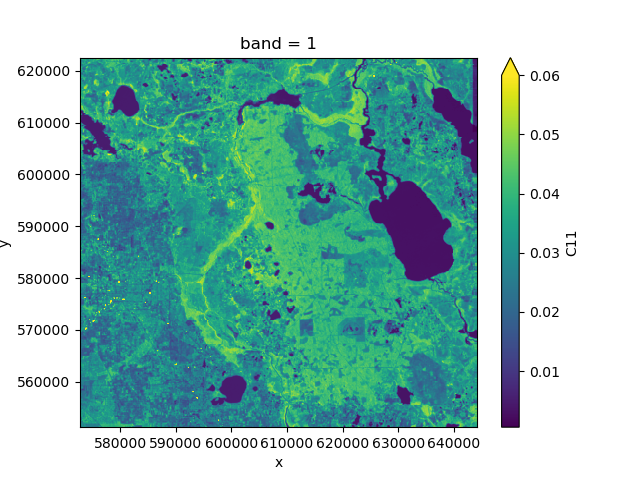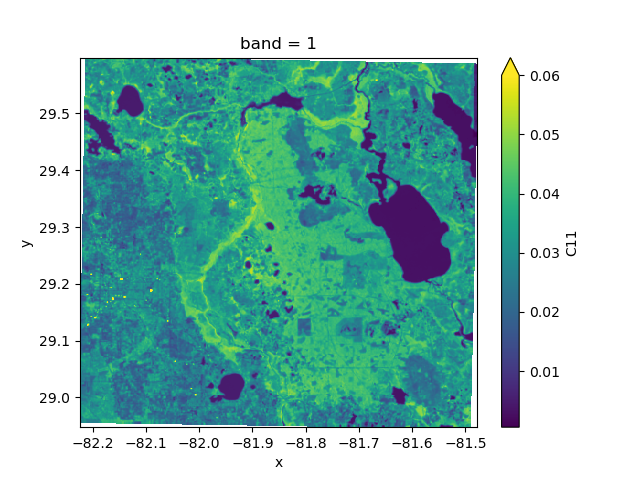# 5. Projections¶

nd handles geographic projections with rasterio. Projection information is usually stored in the metadata attributes crs and transform, but different standards exist.

All functionality related to coordinate systems and projections is contained in the module nd.warp. You can extract the coordinate reference system of a dataset using nd.warp.get_crs():

>>> from nd.warp import get_crs
>>> get_crs(ds)
CRS.from_epsg(3086)


The returned object is always of type rasterio.crs.CRS.

Similarly, the coordinate transformation can be extracted using nd.warp.get_transform():

>>> from nd.warp import get_transform
>>> get_transform(ds)
Affine(178.27973915722524, 0.0, 572867.3883891336,
0.0, -178.27973915722524, 622453.9641249835)


which is an affine.Affine object and represents the mapping from image coordinates to projection coordinates.

Additionally, a dataset contains the coordinates of the x and y axes in ds.coords['x'] and ds.coords['y']. The transform object and the coordinate arrays represent the same information.

## 5.1. Reprojecting to a different CRS¶

You can reproject your dataset to a different coordinate system using nd.warp.Reprojection. For example, the following code will reproject your dataset into Web Mercator (EPSG:3857):

>>> from nd.warp import Reprojection, get_crs
>>> get_crs(ds)
CRS.from_epsg(3086)
>>> proj = Reprojection(crs='EPSG:3857')
>>> ds_reprojected = proj.apply(ds)
>>> get_crs(ds_reprojected)
CRS.from_epsg(3857)

>>> from nd.io import open_dataset
>>> ds = open_dataset('data/C2.nc')
>>> ds.C11.mean('time').plot(vmax=0.06)>>> from nd.warp import Reprojection
>>> epsg4326 = Reprojection(crs='epsg:4326')
>>> proj = epsg4326.apply(ds)
>>> proj.C11.mean('time').plot(vmax=0.06)Reprojection() lets you specify many more options, such as the desired extent and resolution.

When reprojecting a dataset this way, nd will also add coordinate arrays lat and lon to the result which contains the latitude and longitude values at a number of tie points, irrespective of the projection. Storing these arrays alongside the projection information allows GIS software to correctly display the data.